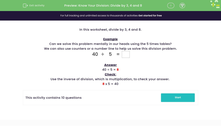# Know Your Division: Divide by 3, 4 and 8

In this worksheet, students divide by 3, 4 and 8.Key stage:  KS 2

Curriculum topic:   Number: Multiplication and Division

Curriculum subtopic:   Use Multiplication/Division Facts (3, 4 and 8)

Difficulty level:#### Worksheet Overview

In this worksheet, divide by 3, 4 and 8.

Example

Can we solve this problem mentally in our heads using the 5 times tables?

We can also use counters or a number line to help us solve this division problem.

 40 ÷ 5 =

40 ÷ 5 = 8

Check:

Use the inverse of division, which is multiplication, to check your answer.

8 x 5 = 40

### What is EdPlace?

We're your National Curriculum aligned online education content provider helping each child succeed in English, maths and science from year 1 to GCSE. With an EdPlace account you’ll be able to track and measure progress, helping each child achieve their best. We build confidence and attainment by personalising each child’s learning at a level that suits them.

Get started